# Inverting Operational Amplifier | Inverting Op Amp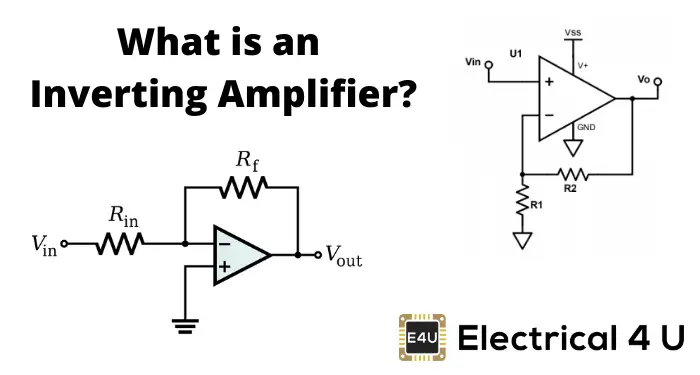## What is an Inverting Amplifier?

An inverting amplifier (also known as an inverting operational amplifier or an inverting op-amp) is a type of operational amplifier circuit which produces an output which is out of phase with respect to its input by 180o.

This means that if the input pulse is positive, then the output pulse will be negative and vice versa. The figure below shows an inverting operational amplifier built by using an op-amp and two resistors.

Here we apply the input signal to the inverting terminal of the op-amp via the resistor Ri. We connect the non-inverting terminal to ground. Further, we provide the feedback necessary to stabilize the circuit, and hence to control the output, through a feedback resistor Rf.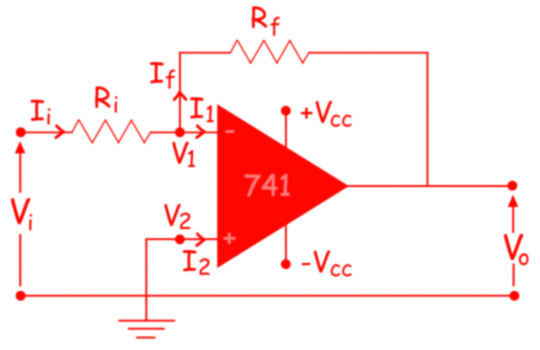Mathematically the voltage gain offered by the circuit is given as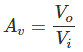Where,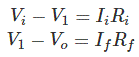However, we know that an ideal op amp has infinite input impedance due to which the currents flowing into its input terminals are zero i.e. I1 = I2 = 0. Thus, Ii = If. Hence,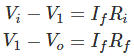We also know that in an ideal op amp the voltage at inverting and non-inverting inputs are always equal.

As we have grounded the non-inverting terminal, zero voltage appears at the non – inverting terminal. That means V2 = 0. Hence, V1 = 0, also. So, we can write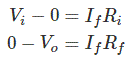From, above two equations, we get,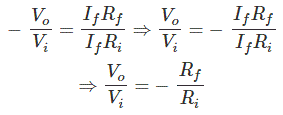The voltage gain of the inverting operational amplifier or inverting op amp is,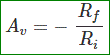This indicates that the voltage gain of the inverting amplifier is decided by the ratio of the feedback resistor to the input resistor with the minus sign indicating the phase-reversal. Further, it is to be noted that the input impedance of the inverting amplifier is nothing but Ri.

Inverting amplifiers exhibit excellent linear characteristics which make them ideal as DC amplifiers. Moreover, they are often used to convert input current to the output voltage in the form of Transresistance or Transimpedance Amplifiers. Further, these can also be used in audio mixers when used in the form of Summing Amplifiers.

Want To Learn Faster? 🎓
Get electrical articles delivered to your inbox every week.
No credit card required—it’s 100% free.